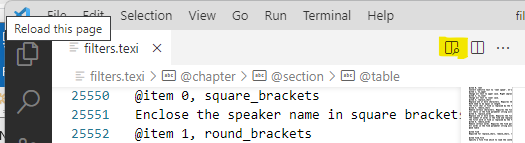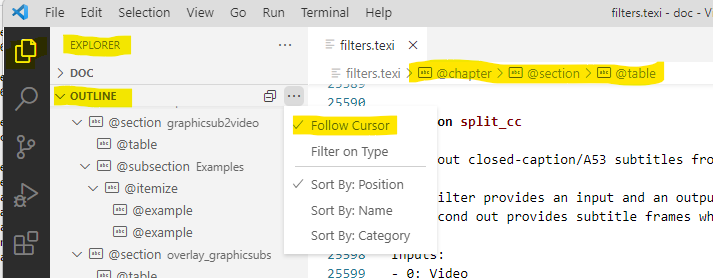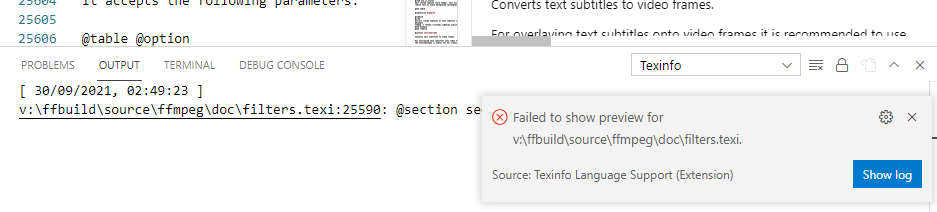{{ message }}

Instantly share code, notes, and snippets.

# softworkz/EditingTexiFiles.md Secret

Last active Sep 30, 2021
Editing .texi files with VSCode on Windows

Compilation of .texi docs is not part of my primary development workflow, and as such it has always been an annoyance to see errors only at a secondary stage when doing platform compilations.

It's not that editing .texi would be a challenging task - at least not when keeping the use of .text features at the same minimal level as most of the existing entries. But when trying to use more texinfo features, even as simple as cross-references (do they resolve properly or not?), this can quickly turn into a trial-and-error game.

Without having an editor that is capable to provide instant preview and feedback about the validity of new entries, developers will often resort to go the easy way just to avoid any additinal hazzle.

## TeX Editors

I've looked through quite a number of ways for nicely editing .texinfo files with preview and error display. Generally, TeX (not LaTeX) is able to process and render .texi files, when there's a texinfo.tex file in the same folder. While this is working at the side of rendering, we still don't have an editing workflow. I have tried quite a number of TeX editors for Windows. Some are limited to LaTeX only, others are more flexible but with each I have tried, there was an important element missing. Some had no side-by-side preview, just creation of a full PDF, some weren't able to relate rendering erors to the document lines where the erorrs were located, and another one provided a nice side-by-side preview with synchronization of scroll position and even synchronized text selection between source and preview, but it didn't have a generated TOC and the text editor was minimal without any highlighting or tag completion.

## VSCode

There is a texinfo plugin for VS Code (https://savannah.nongnu.org/p/vscode-texinfo), but I couldn't get it to work on Windows as it requires the texinfo (https://savannah.gnu.org/p/texinfo) tools to be available. There are just a number of older versions available as Windows binaries and none of them did really work. Recent versions of texinfo seem to have switched to perl and that perl code seems to have some hard-coded Linux paths.

I found one solution though to get it working:

Running the Linux version of the texinfo tool (makeinfo) via WSL (Ubuntu). The execution of Linux commands from Windows couldn't be easier (just try `wsl uname -a`). The only problem are the different path names, for which I've come up with a (pretty ugly) batch script:

```@echo off

set cmd=%*

set cmd=%cmd:a:\=/mnt/a/%
set cmd=%cmd:b:\=/mnt/b/%
set cmd=%cmd:c:\=/mnt/c/%
set cmd=%cmd:d:\=/mnt/d/%
set cmd=%cmd:e:\=/mnt/e/%
set cmd=%cmd:f:\=/mnt/f/%
set cmd=%cmd:g:\=/mnt/g/%
set cmd=%cmd:h:\=/mnt/h/%
set cmd=%cmd:i:\=/mnt/i/%
set cmd=%cmd:j:\=/mnt/j/%
set cmd=%cmd:k:\=/mnt/k/%
set cmd=%cmd:l:\=/mnt/l/%
set cmd=%cmd:m:\=/mnt/m/%
set cmd=%cmd:n:\=/mnt/n/%
set cmd=%cmd:o:\=/mnt/o/%
set cmd=%cmd:p:\=/mnt/p/%
set cmd=%cmd:q:\=/mnt/q/%
set cmd=%cmd:r:\=/mnt/r/%
set cmd=%cmd:s:\=/mnt/s/%
set cmd=%cmd:t:\=/mnt/t/%
set cmd=%cmd:u:\=/mnt/u/%
set cmd=%cmd:v:\=/mnt/v/%
set cmd=%cmd:w:\=/mnt/w/%
set cmd=%cmd:x:\=/mnt/x/%
set cmd=%cmd:y:\=/mnt/y/%
set cmd=%cmd:z:\=/mnt/z/%

set cmd=%cmd:\=/%

wsl makeinfo %cmd% > tmp_out.txt  2>&1

set err=%ERRORLEVEL%

if %ERRORLEVEL% == 0 (
type tmp_out.txt
exit /b 0
)

set /p res=<tmp_out.txt

set res=%res:/mnt/a/=a:\%
set res=%res:/mnt/b/=b:\%
set res=%res:/mnt/c/=c:\%
set res=%res:/mnt/d/=d:\%
set res=%res:/mnt/e/=e:\%
set res=%res:/mnt/f/=f:\%
set res=%res:/mnt/g/=g:\%
set res=%res:/mnt/h/=h:\%
set res=%res:/mnt/i/=i:\%
set res=%res:/mnt/j/=j:\%
set res=%res:/mnt/k/=k:\%
set res=%res:/mnt/l/=l:\%
set res=%res:/mnt/m/=m:\%
set res=%res:/mnt/n/=n:\%
set res=%res:/mnt/o/=o:\%
set res=%res:/mnt/p/=p:\%
set res=%res:/mnt/q/=q:\%
set res=%res:/mnt/r/=r:\%
set res=%res:/mnt/s/=s:\%
set res=%res:/mnt/t/=t:\%
set res=%res:/mnt/u/=u:\%
set res=%res:/mnt/v/=v:\%
set res=%res:/mnt/w/=w:\%
set res=%res:/mnt/x/=x:\%
set res=%res:/mnt/y/=y:\%
set res=%res:/mnt/z/=z:\%

set res=%res:/=\%

echo %res%>&2

exit /b %err%```

## Usage

See documentation of Texinfo extension: https://nongnu.org/vscode-texinfo/manual/

Everything (preview, toc, errors) is updated when saving the .texi document.

### Preview

• Hit button to open preview:### TOC

• Open Explorer bar and collapse 'DOC' to view the TOC of the .texi file.
• Activate "Follow Cursor"
• Use breadcrumps at the top of the editor### ErrorsCTRL-Click on the line in the error output works, erorrs are not shown in the 'Problems' list (don't know why).

This file contains bidirectional Unicode text that may be interpreted or compiled differently than what appears below. To review, open the file in an editor that reveals hidden Unicode characters. Learn more about bidirectional Unicode characters
 @echo off set cmd=%* set cmd=%cmd:a:\=/mnt/a/% set cmd=%cmd:b:\=/mnt/b/% set cmd=%cmd:c:\=/mnt/c/% set cmd=%cmd:d:\=/mnt/d/% set cmd=%cmd:e:\=/mnt/e/% set cmd=%cmd:f:\=/mnt/f/% set cmd=%cmd:g:\=/mnt/g/% set cmd=%cmd:h:\=/mnt/h/% set cmd=%cmd:i:\=/mnt/i/% set cmd=%cmd:j:\=/mnt/j/% set cmd=%cmd:k:\=/mnt/k/% set cmd=%cmd:l:\=/mnt/l/% set cmd=%cmd:m:\=/mnt/m/% set cmd=%cmd:n:\=/mnt/n/% set cmd=%cmd:o:\=/mnt/o/% set cmd=%cmd:p:\=/mnt/p/% set cmd=%cmd:q:\=/mnt/q/% set cmd=%cmd:r:\=/mnt/r/% set cmd=%cmd:s:\=/mnt/s/% set cmd=%cmd:t:\=/mnt/t/% set cmd=%cmd:u:\=/mnt/u/% set cmd=%cmd:v:\=/mnt/v/% set cmd=%cmd:w:\=/mnt/w/% set cmd=%cmd:x:\=/mnt/x/% set cmd=%cmd:y:\=/mnt/y/% set cmd=%cmd:z:\=/mnt/z/% set cmd=%cmd:\=/% wsl makeinfo %cmd% > tmp_out.txt 2>&1 set err=%ERRORLEVEL% if %ERRORLEVEL% == 0 ( type tmp_out.txt exit /b 0 ) set /p res=&2 exit /b %err%
to join this conversation on GitHub. Already have an account? Sign in to comment